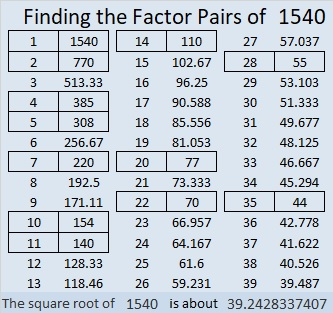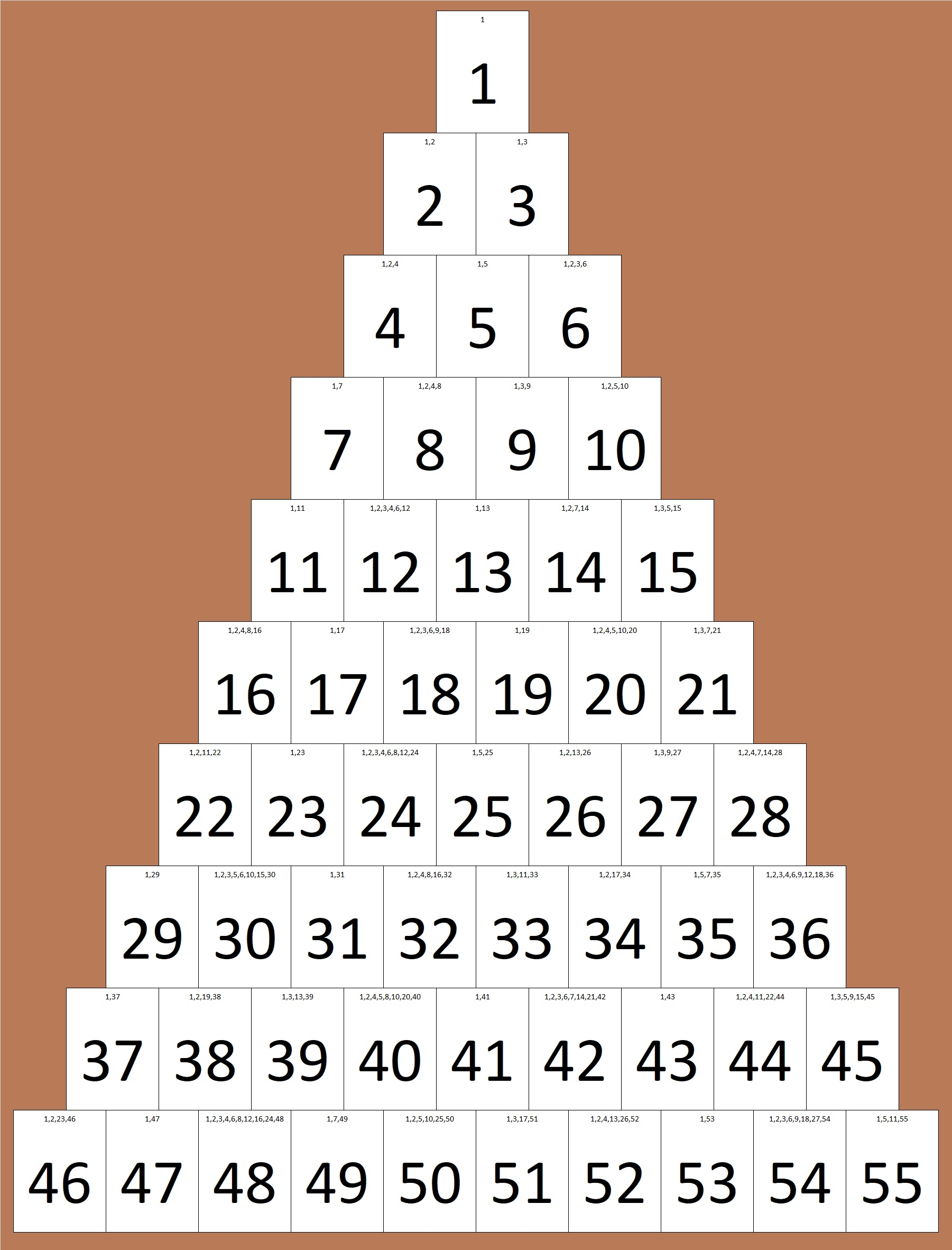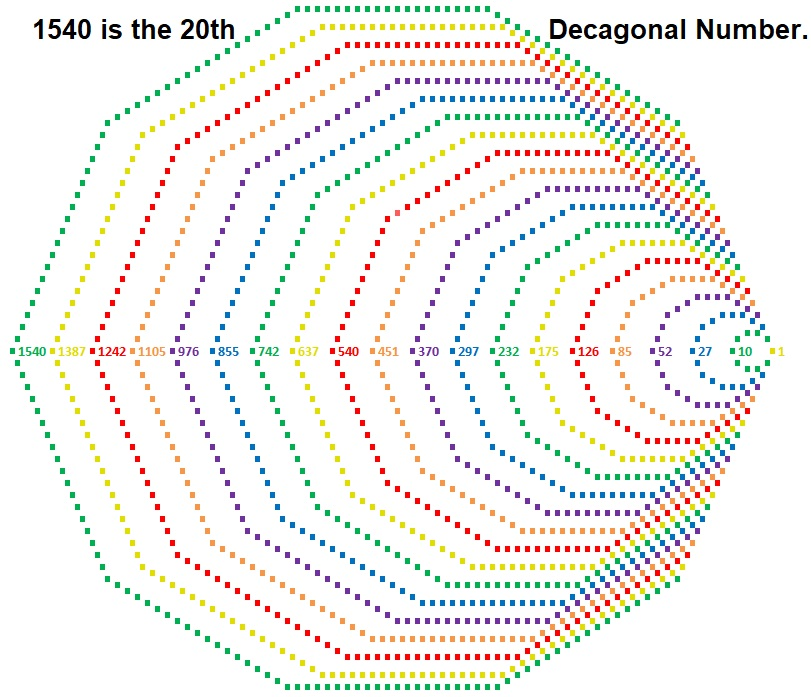# How Much of My \$1540 Will The Taxman Get?

Contents

### The Taxman Game

You might think that the picture below is just the numbers from 1 to 55 arranged in a 5×11 rectangle.

It’s really PLAY MONEY, one bill in each denomination from 1 to 55. If you add up the total value of all this play money, you will get 1540 because the sum of the numbers from 1 to 55 is 1540.Most of that 1540 in play money can be yours to keep if you outsmart THE TAXMAN.

You can take any bill that you want, but The Taxman has to get paid every time you do. The Taxman will get ALL the available factors of the bill you take. If there isn’t at least one bill on hand that is a factor of your chosen bill, then you can’t take that bill. When none of the bills left are factors of any of the other bills that are left, The Taxman gets ALL the remaining bills. The value of those bills could add up quickly, and that’s how The Taxman might outsmart you.

You can print this excel file to play the Taxman game: Taxman & 1537-1544 It has all the play money bills from 1 to 100, and all the factors are typed on the top of the bills!

The factors at the top of each bill make it possible to play the game even if you don’t know how to multiply or divide yet. My granddaughter learned to play the game sometime around her eighth birthday. Before tackling 55 bills, she learned what to do for a smaller number of bills. I told her:
If 1 is the only bill, The Taxman wins.
If 1, 2 are the only bills, you take the 2, The Taxman gets the 1, and you win.
If 1, 2, 3 are the only bills, the best you can do is a draw (1 + 2 = 3),
But if there are at least four bills, it is possible to win every time.

She played the game over and over adding one more bill to the game each time she played. She enjoyed it very much and played it over 20 times until bedtime required her to stop. Each time she played we talked about how she would arrange the cards in a rectangle. For example, if she used cards 1 – 6, she would look at the factors of 6 and decide on a 2 × 3 rectangle or a 1 × 6 rectangle.

Knowing I wanted to write this post about the 55th triangular number (1540), I had her jump to using 55 bills the next day, but I helped her with it a little. We didn’t want to just win the game, we wanted to make it so The Taxman would get as little money as possible. Here is the final order of what we took and what The Taxman got.Laying it out like that made it easy to have do-overs when needed.

### A Way to Keep Score When Playing Taxman:

When it was time to score the game, instead of adding up all the numbers to see if we took more than The Taxman, we found smaller sums that equaled as many of our choices as we could as illustrated below. For example,  47 + 6 = 53, so there was no need to add 47 + 6 to The Taxman’s total or to add 53 to ours.I found it enjoyable finding all those sums, but it isn’t necessarily easy for a child to do. After arranging all those sums on the table, we were able to see that we scored
35 + 39 + 38 + 12 + 30 + 50 + 24 + 48 + 42 = 318 more than The Taxman did.

We can use algebra to figure out exactly how much of our 1540 in play money we get to keep.
If The Taxman got X of the play money, we kept X + 318 of it, and
X + X + 318 = 1540,
2X + 318 = 1540,
2X + 318 – 318 = 1540 – 318,
2X = 1222,
X = 611, that’s what The Taxman got.
We kept 611 + 318 = 929 of our play money.

What percentage of the play money did The Taxman get?
611/1540 ≈ 0.40 which is 40%. Wow, that’s a lot!

### Factors of 1540:

• 1540 is a composite number.
• Prime factorization: 1540 = 2 × 2 × 5 × 7 × 11, which can be written 1540 = 2² × 5 × 7 × 11.
• 1540 has at least one exponent greater than 1 in its prime factorization so √1540 can be simplified. Taking the factor pair from the factor pair table below with the largest square number factor, we get √1540 = (√4)(√385) = 2√385.
• The exponents in the prime factorization are 2, 1, 1, and 1. Adding one to each exponent and multiplying we get (2 + 1)(1 + 1)(1 + 1)(1 + 1) = 3 × 2 × 2 × 2 = 24. Therefore 1540 has exactly 24 factors.
• The factors of 1540 are outlined with their factor pair partners in the graphic below.### Factor Cake for 1540:

1540 has lots of prime factors with 11 being the greatest so it makes a lovely factor cake:### What Kind of Shape Is 1540 in?

I know that 1540 = 55×56÷2 so, 1540 is the 55th triangular number. Guess what? Since 55 is also a triangular number (55 = 10×11÷2), we could have arranged the play money bills in a triangle like this instead of a 5 × 11 rectangle.1540 is not only the 55th triangular number, but it is also the sum of the first 20 triangular numbers:

1+3+6+10+15+21+28+36+45+55+66+78+91+105+120+136+153+171+190+210 = 1540.

If you stack those twenty triangular numbers from smallest to biggest, you will get something shaped like a tetrahedron. That’s why we say that 1540 is the 20th tetrahedral number. We can also use a formula for the 20th tetrahedral number:
20(21)(22)/6 = 1540.

1540 is also the 28thhexagonal number. (All hexagonal numbers are also triangular numbers. That’s why it’s the 2(28)-1 = 55th triangular number.) It is the 28th hexagonal number because 2(28²) – 28 = 1540.1540 is the 20th decagonal number because 4(20²) – 3(20) = 1540.I didn’t make a visual, but 1540 is also the19th centered nonagonal number.
That’s because
3 × 19 = 57 and (57 – 2)(57 – 1)/2 = 1540.

1540 is also the hypotenuse of a Pythagorean triple triangle:
924-1232-1540 which is (3-4-5) times 308.

1540 is quite the shape-shifter number, isn’t it!

This site uses Akismet to reduce spam. Learn how your comment data is processed.